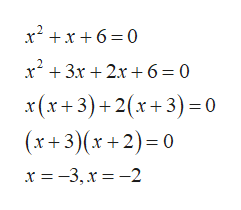Find the domain of function?f(x)=x+2/x2+x+6

Question

Find the domain of function?

f(x)=x+2/x2+x+6

Step 1

DOMAIN is the set of all values of x for which function is not defined.

To find the domain of the function, put the denominator of the function equal to zero.

Function:

Step 2

Put denominator equal to zero that is;

Step 3

Now, solve the equation by &nbs...help_outlineImage Transcriptionclosex2x6 0 3x2 +6 = 0 x(x+3)+2x3) 0 (x+3) (x+2) 0 x =-3, x =-2 fullscreen

Want to see the full answer?

See Solution

Want to see this answer and more?

Our solutions are written by experts, many with advanced degrees, and available 24/7

See Solution
Tagged in

Other